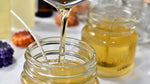# Calculating how much wax to use when making a candleOne of the most important things when making candles is making sure you’re measuring the correct amount of materials – if you use too much you’ll be wasting money with leftovers, use too little and you’ll not have enough to make the desired amount of products.

When it comes to measuring wax it’s best to get it right first time, this is the biggest part of a candle and it’s imperative you get the measurements right so even if you’re using a new container, you’ll be able to work out how much wax to use.

One important thing to know when measuring wax is that although it turns to liquid when melted it is not the same weight as water as a lot of people think (this is the same with fragrance oils too, but we’ll get to that in another article!), the wax is less dense than water so weighs less.

Candle Wax is less dense than water as it’s composed of carbon & hydrogen atoms, water is composed of oxygen & hydrogen atoms. Oxygen atoms are heavier & smaller than carbon atoms. This is why wax floats on water.

Generally, paraffin wax and soy candle wax is 90% the density of water, 900 kg/m3

Calculating the volume of a container using water

The simplest way to calculate the volume of a container is to use water and is great for all shapes of container…

Using a measuring jug:

Fill your container to the level you’ll want your final candle to be and then empty the water into a measuring jug and note down the measurement in ml.

Weighing using scales:

Place your empty container on your scales and ‘tare’ or ‘zero’ your scales, you can then remove your container and fill with water to the line you will want your wax to come to, then weigh on the scales, the weight on the scales will represent both grams and ml – one millilitre of water is one gram.

Calculating how much wax you’ll need

Once you’ve worked out the volume of grams or millilitre then you’ll be able to work out how much wax you’ll need to fill your container.

Simply write down the volume of your container and then multiply it by 0.9

This 0.9 factor takes into account the lower density of wax (900 kg/m3) and the calculated figure will give the volume of wax needed in Grams for your container – remember this doesn’t take into account any fragrance you will be using, you will need to ensure you make an allowance for the fragrance in your final calculations.

Example:

If you have a 20cl Karen glass the full volume of this is 200ml/g but, you’ll want to have a wax level just under 1cm from the top so we’ve filled it with 150 ml/g of water.

Calculation: 150 x 0.9 = 135 grams
150 (the level of water in a container) x 0.9 (converts density of water to the weight of wax) = 135 (grams of wax you’ll need)

Now you can easily calculate the amount of wax you’ll need for multiple containers, just multiply the total [135g] by the number of containers, for example, 12 containers – 135 x 12 = 1620 grams.

Note that these calculations don’t include fragrance, this should be measured out in grams, a quick calculation for a 10% fragrance load in the single vessel would be: 135 x 0.1 = 13.5 grams (where 0.1 represents 10% of the original number)

So the calculation for wax with a fragrance load of 10% would be:

135 x 0.1 =13.5 then remove this from the total wax: 135 – 13.5 = 121.5